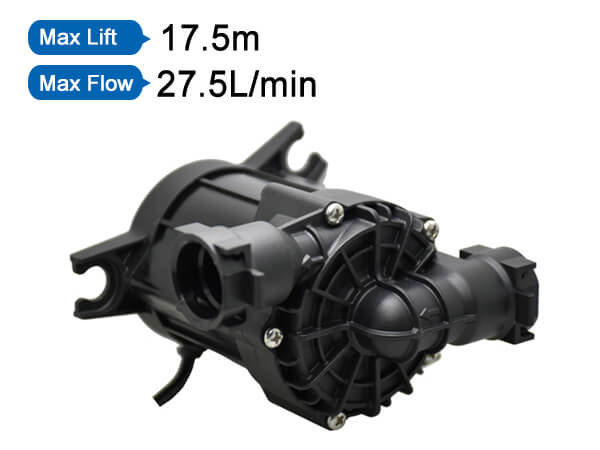### Shenpeng Electronics

Water Pump to provide solutions

+86-769-81868638

# The basic parameters of the pump

Published:2021-10-14The basic parameters of the main performance of the pump are as follows:

1. Flow rate Q Flow rate is the amount of liquid (volume or mass) delivered by the pump in a unit time. The volume flow is expressed by Q, and the unit is: m3/s, m3/h, l/s, etc. The mass flow rate is expressed by Qm, and the unit is: t/h, kg/s, etc. The relationship between mass flow and volume flow is: Qm=ρQ where 　ρ——the density of the liquid (kg/m3, t/m3), and clean water at room temperature ρ=1000kg/m3.

2. Head H Head is the increase in energy of the unit weight of liquid pumped by the pump from the pump inlet (pump inlet flange) to the pump outlet (pump outlet flange). That is, the effective energy of a Newtonian liquid obtained by the pump. Its unit is N·m/N=m, that is, the height of the liquid column of the pumped liquid, and it is often referred to as meter for short.

3. Rotation speed n Rotation speed is the number of revolutions of the pump shaft per unit time, expressed by the symbol n, and the unit is r/min.

4. The cavitation margin.

NPSH cavitation margin is also called net positive tip, which is the main parameter indicating cavitation performance. The NPSH has been expressed by Δh in China.

5. Power and efficiency The power of the pump usually refers to the input power, that is, the power on the shaft of the prime mover, so it is also called the shaft power, which is represented by P; the effective power of the pump is also called the output power, which is represented by Pe. Diaphragm pump is the effective energy obtained in the pump from the liquid delivered from the pump per unit time. Because the head refers to the effective energy obtained from the pump by the unit heavy liquid output by the pump, the product of the head, mass flow rate and gravitational acceleration is the effective energy obtained from the liquid output from the pump per unit time-that is, the pump Effective power: Pe=ρgQH(W)=γQH(W) where ρ——the density of the liquid conveyed by the pump (kg/m3); γ——the gravity of the liquid conveyed by the pump (N/m3); Q——the pump’s Flow rate (m3/s); H——pump head (m); g——gravitational acceleration (m/s2). The difference between the shaft power P and the effective power Pe is the power loss in the pump, and its magnitude is measured by the efficiency of the pump. The efficiency of the pump is the ratio of the effective power to the shaft power, expressed by η.

News
service telephone:0769-81868638